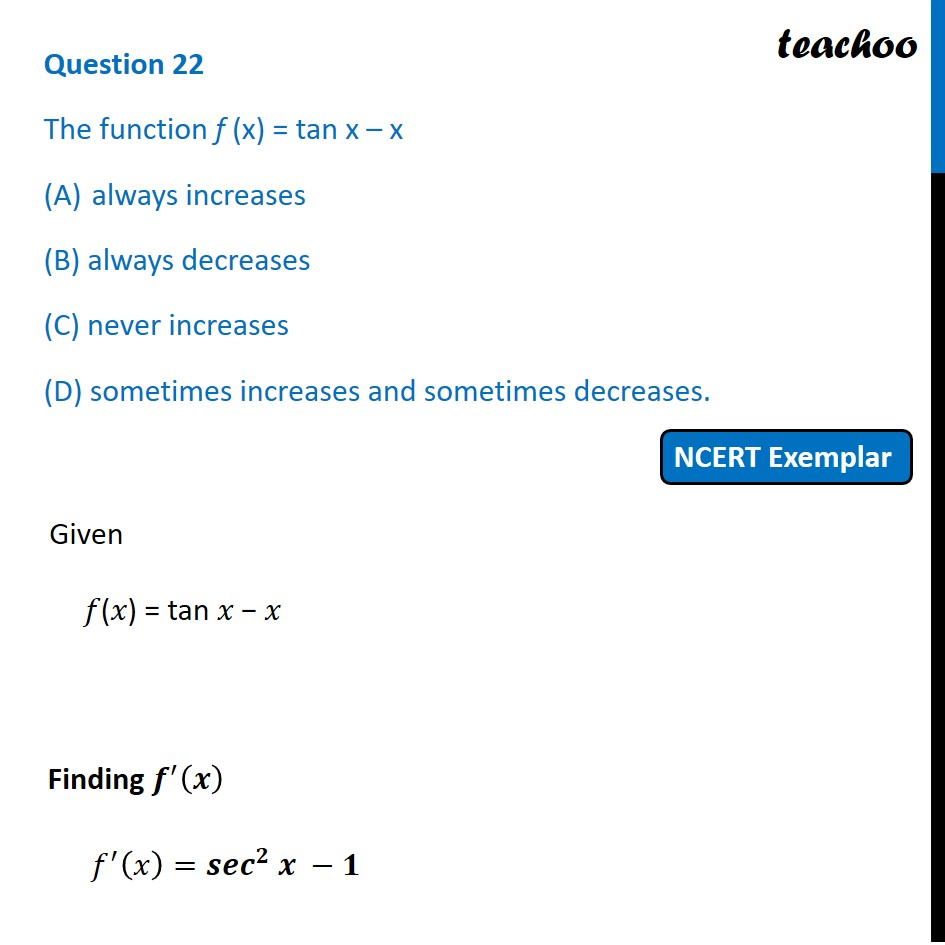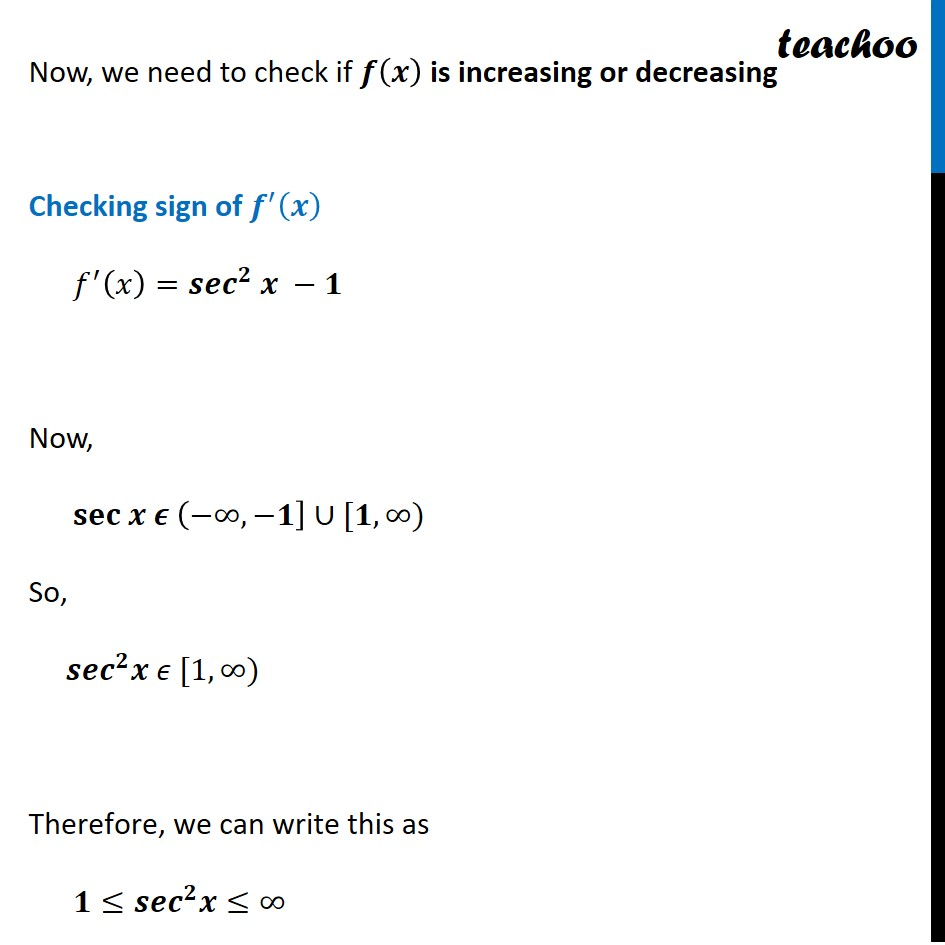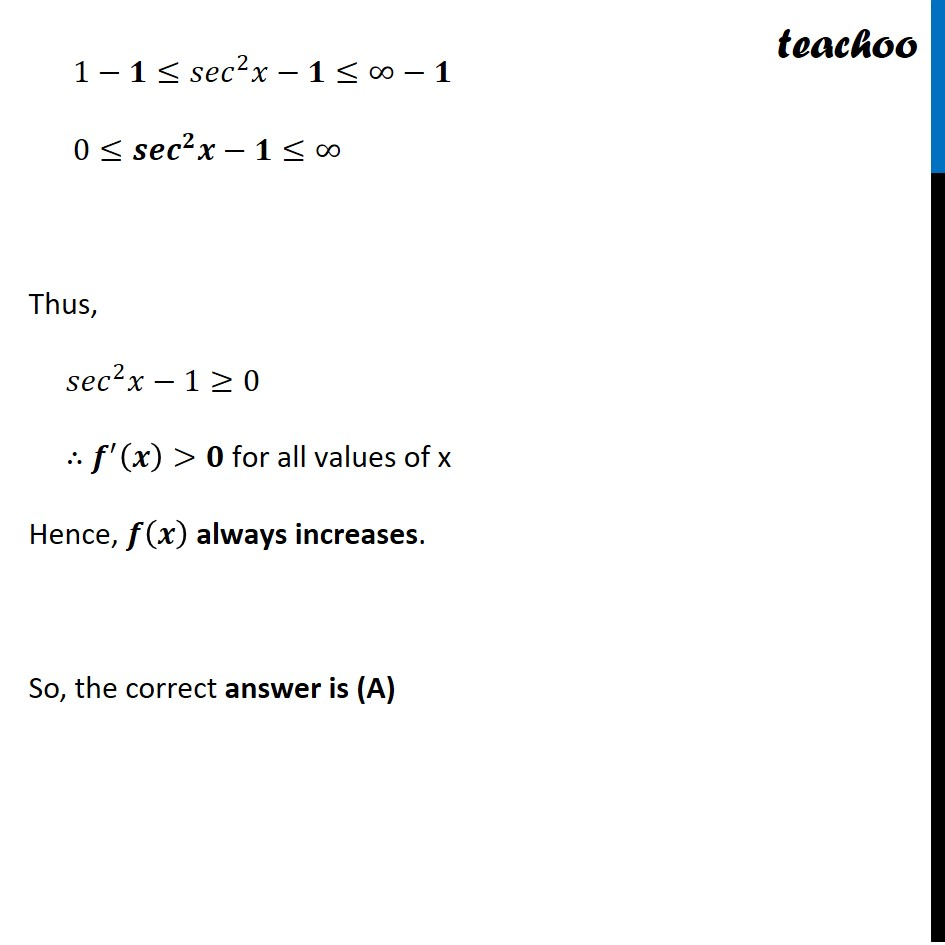NCERT Exemplar - MCQs

Chapter 6 Class 12 Application of Derivatives
Serial order wise

## (D) sometimes increases and sometimes decreases.

This question is similar to Question 32 CBSE Class 12 Sample Paper 2021 Boards - Application of DerivativesLearn in your speed, with individual attention - Teachoo Maths 1-on-1 Class

### Transcript

Question 9 The function f (x) = tan x – x always increases (B) always decreases (C) never increases (D) sometimes increases and sometimes decreases. Given 𝑓(𝑥) = tan 𝑥 − 𝑥 Finding 𝒇^′ (𝒙) 𝑓^′ (𝑥)=〖𝒔𝒆𝒄〗^𝟐 𝒙 −𝟏 Now, we need to check if 𝒇(𝒙) is increasing or decreasing Checking sign of 𝒇^′ (𝒙) 𝑓^′ (𝑥)=〖𝒔𝒆𝒄〗^𝟐 𝒙 −𝟏 Now, 𝐬𝐞𝐜 𝒙 𝝐 (−∞,−𝟏]∪[𝟏,∞) So, 〖𝒔𝒆𝒄〗^𝟐 𝒙 𝜖 [1,∞) Therefore, we can write this as 𝟏≤〖𝒔𝒆𝒄〗^𝟐 𝒙≤∞ 1−𝟏≤〖𝑠𝑒𝑐〗^2 𝑥−𝟏≤∞−𝟏 0≤〖𝒔𝒆𝒄〗^𝟐 𝒙−𝟏≤∞ Thus, 〖𝑠𝑒𝑐〗^2 𝑥−1≥0 ∴ 𝒇^′ (𝒙)>𝟎 for all values of x Hence, 𝒇(𝒙) always increases. So, the correct answer is (A)